# Test: Communication System

## 26 Questions MCQ Test GATE ECE (Electronics) 2023 Mock Test Series | Test: Communication System

Description
Attempt Test: Communication System | 26 questions in 75 minutes | Mock test for Electronics and Communication Engineering (ECE) preparation | Free important questions MCQ to study GATE ECE (Electronics) 2023 Mock Test Series for Electronics and Communication Engineering (ECE) Exam | Download free PDF with solutions
QUESTION: 1

### The DSB-SC signal recevied at the output of a channel is given as S(t) = 2 cos2π(103 - 0.5). cos2π(106 - 1). Determine the group delay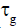and phase delay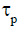introduced by the channel.

Solution:

For an amplitude modulated signal
the delay in carrier frequency
component gives phase delay and
delay in baseband (message)
signal gives the group delay.
{Reference: Page No 98, Simon
Haykin, common system 3rd
edition}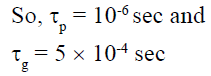QUESTION: 2

### Which of the following is incorrect about hilbert-transform?

Solution:

xh (t) is delayed version by -900 of
x(t)

QUESTION: 3

### An amplitude modulated signal is given as s(t) = 14 cos8000πt + 20 cos10000πt + 14 cos12000πt. Determine the modulation depth.

Solution: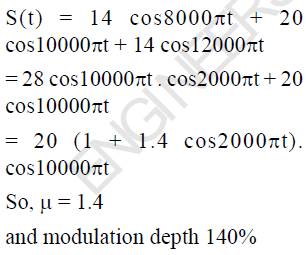QUESTION: 4

An amplitude modulated signal is given as S(t) = 10 (2 + cos2π x 104t) cos2π x 107t. If the carrier
frequency component is suppresed before transmission, then determine the percentage power
saved.

Solution: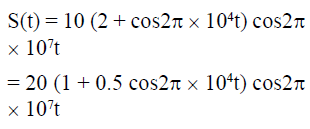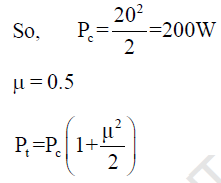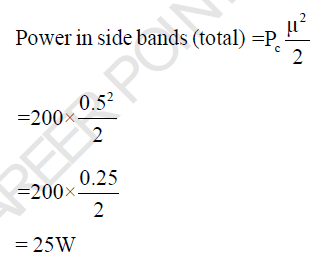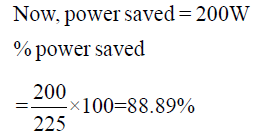QUESTION: 5

Suppose that non linear device (NLD) is available for which the output voltage V0 and input voltage Vi are related by V0(t) = a1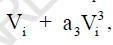where a1 and a3 are constants. Let Vi = m (t) + cos2πfct and a1 = 1, a2 = 1. To generate a DSB-SC signal having carrier frequency is 5MHz, this NLD is followed by a BPF of appropriate centre frequency and band width. Determine fc

Solution: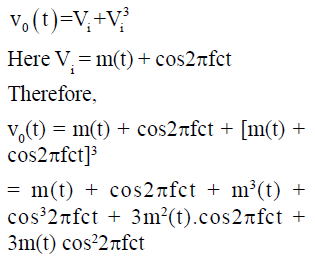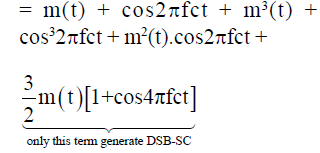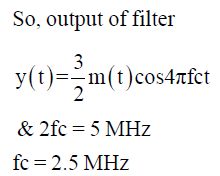QUESTION: 6

Consider a square law device having input voltage Vi and output voltage V0 are related as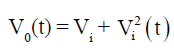This SLD is used to generate an standard AM with a carrier frequency of 3MHz, as shown in the circuit below.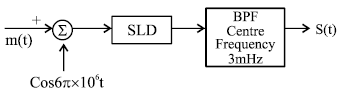Determine the maximum bandwidth of message signal m(t) so that spectrum of s(t) is nonoverlapping.

Solution: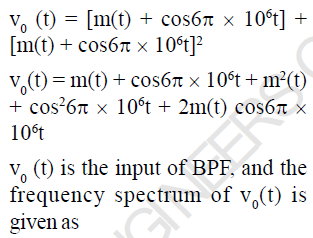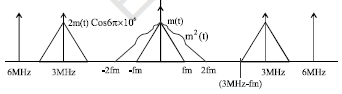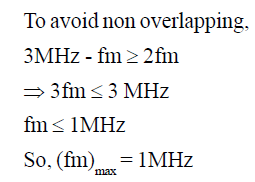i.e the maximum bandwidth of
message signal may be 1MHz

QUESTION: 7

A message signal m(t) = 4 cos2πx 104 t is used to generate an FM signal. Determine the minimum
value of (Δf)max so that modulation efficiency is 100%.

Solution:

M(t) = 4cos2π x 104t
Here Am = 4, fm = 10 KHz,
Modulation efficiency is 100%

when βf = 2.4, 5.5, 8.6-----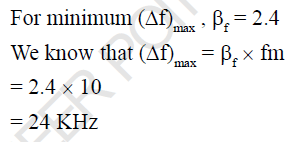QUESTION: 8

A phase-modulated signal is given as S(t) = 30 cos[2πx108t + 100 cos2πx104t]. Determine the
transmission bandwidth required according to carson’s approximation.

Solution: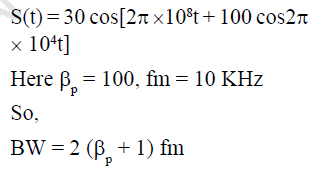= 2 (100 + 1) x 10
= 20 x 101
= 2020 KHz
= 2.02 MHz

QUESTION: 9

Consider an FM signal, S(t) = 10 cos[2π x107t + 10 sin2π x103t]. Determine Kp/Kf if the above modulated signal is assumed phase-modulated signal.

Solution: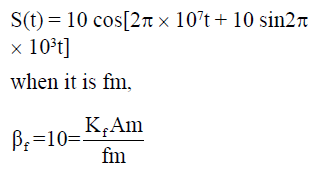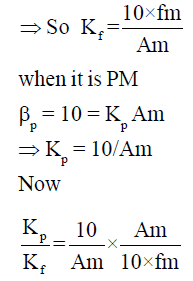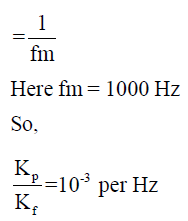QUESTION: 10

The following message signal m(t) is given to both frequency modulator and phase modulator. If
the maximum phase deviation of both FM and PM is same, determine the Kf/Kp.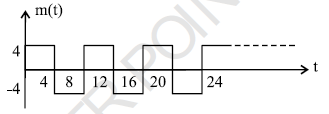Solution: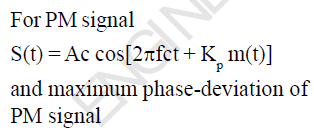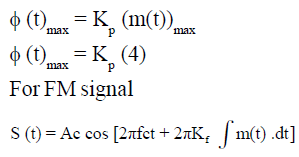and maximum phase-deviation of
FM signal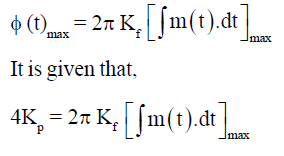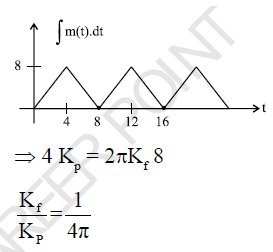QUESTION: 11

Consider a sine wave of frequency 2 KHz and peak amplitude AM. This signal is applied to a delta modulator of step-size 0.4 Volt and sampling period 3.18 x 10-6 sec. What is the maximum power that
may be transmitted without slopeoverload distortion?

Solution: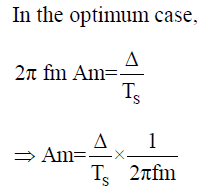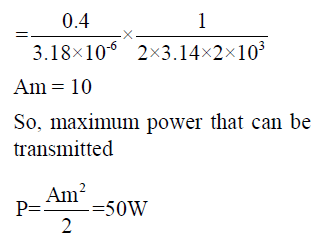QUESTION: 12

A PCM system uses an uniform quantizer followed by a 7-bit binary encoder. The bit rate of the system is equal to 50x106 bits/ second. Determine the output signal to quantization noise ratio when a full-load sinusoidal modulating signal of frequency 1MHz is applied to the input.

Solution:

(SNR) = (6n + 1.8) dB
= 6 x 7 + 1.8
= 43.5 dB

QUESTION: 13

An analog signal is sampled, quantized and encoded into a binary PCM system. The specifications of the PCM system include the following: Sampling role = 8 KHz Number of representation levels = 64
Determine the minimum bandwidth required if PCM wave is transmitted over a baseband channel.

Solution: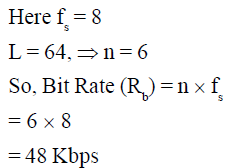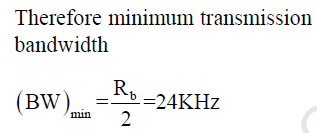QUESTION: 14

A binary PCM system uses pulse P(t) to transmit symbol 1 and - P(t) to transmit symbol 0. The additive noise at the reciever input is white and Gaussian, with zero mean and power spectral density
10-10 W/Hz. Assuming that symbols 1 and 0 occur with equal probability, determine the probability of error at the reciever output.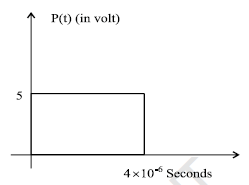Solution:

For binary polar signal the
probability of error is given as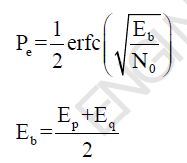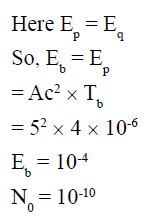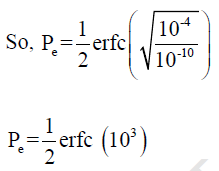QUESTION: 15

A source emits one of four symbols S0, S1, S2 and S3 with probabilities 1/3, 1/6, 1/4 and 1/4 respectively.
The successive symbols emitted by the source are statistically independent. Calculate the entropy
of the source.

Solution: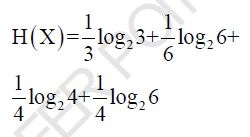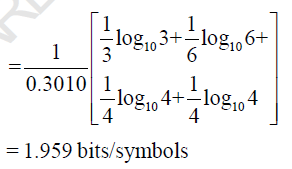QUESTION: 16

Consider a sinusoidal signal with random phase, defined by X(t) =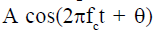where A and fare constants and θ is a random variable that is uniformly distributed over the interval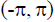Determine the autocorelation function of X (t)

Solution:

The probability function of random
variable is given as,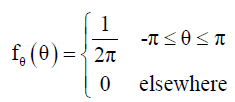Now the auto-corelation function
of X(t)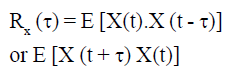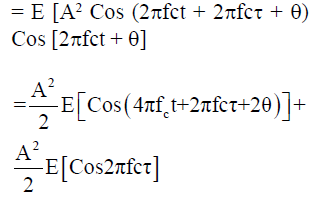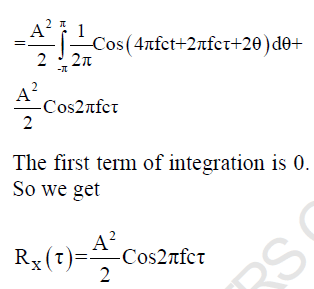QUESTION: 17

The probability density function of a random variable is shown in the figure. Determine P (X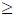0)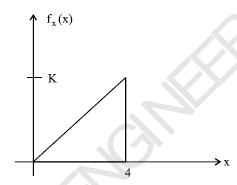Solution:

The probability for X0 is exactly
1. (i.e whole area under density
function curve)

QUESTION: 18

A 1W video signal having bandwidth of 200 MHz is transmitted to a reciver through a cable that has 50 dB loss. If the efficitive two sided noise power spectred density at the receiver is 10-20 Watt/Hz, then the signal to noise ratio at the receiver is

Solution:

PS = 1 W
Since cable loss is 50 dB (105), So
power at the input of reciever
PS = 10-5 Watts
Noise power = 2 x200 x106 x10-20
2, Because of two sided PSD
= 4 x 10-12 Watts
Therefore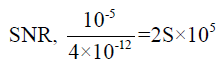QUESTION: 19

A computer puts out binary data at the rate of 56 kbps. The computer output is transmitted using a
baseband binary PAM system that is designed to have a raised cosine spectrum. Determine band width
required if the roll-off factor is 0.5.

Solution:

Bit Rate (Rb) = 56 Kbps
Since it is transmitted by 2-array,
so minimum bandwidth without
raised-cosine pattern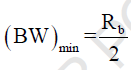and minimum bandwidth with
raised-cosine pattern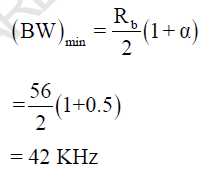QUESTION: 20

A QPSK system transmits binary data at the rate of 2.5 x 106 bit per second. Determine minimum
bandwidth required for the transmission.

Solution: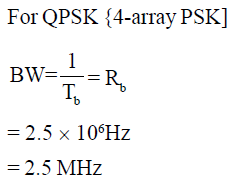QUESTION: 21

For attenuation of high frequencies we should use

Solution:

Since Xc for high frequencies is low, the high frequencies are shunted to ground and are not transmitted.

QUESTION: 22

The pulse shown in the figure, is given as the input of a matched filter. Determine the peak value of
the output of matched filter.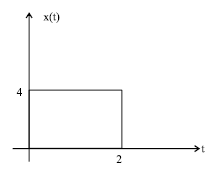Solution: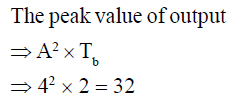QUESTION: 23

A binary random variable X takes the value of 1 with probability 2/3. X is input to a binary symetric
channel with cross-over probability 1/2.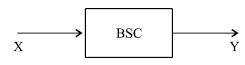Determine the value of H(Y)

Solution: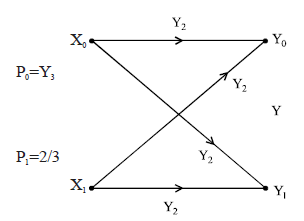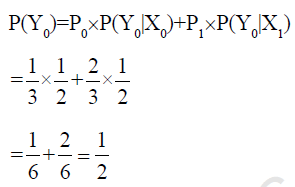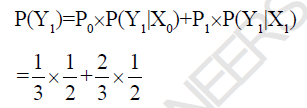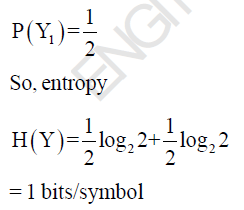QUESTION: 24

A voice-grade channel of the telephone network has a bandwidth of 3.4 KHz calculate the information capacity of the telephone channel for a signal to noise ratio of 30 dB.

Solution:

Capacity of a gaussian channel is
given as,

C = BW log2 (1 + SNR)

Here SNR = 30 dB (1000)
So,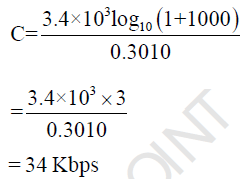QUESTION: 25

Determine the minimum sampling frequency of x(2t), where x(t) = sine (1000t)

Solution:

Minimum sampling frequency of
x(t) is 1000 Hz (1KHz)
So, minimum sampling frequency
of x(2t) is 2KHz

QUESTION: 26

The probability density function of random variable X is given as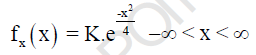Determine the variance (σ2) of random variable X.

Solution:

Given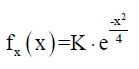Comparing this equation with the
standard gaussian probability
density function
i.e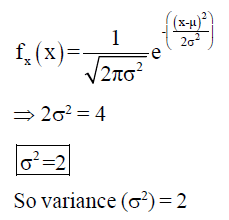Use Code STAYHOME200 and get INR 200 additional OFF Use Coupon Code